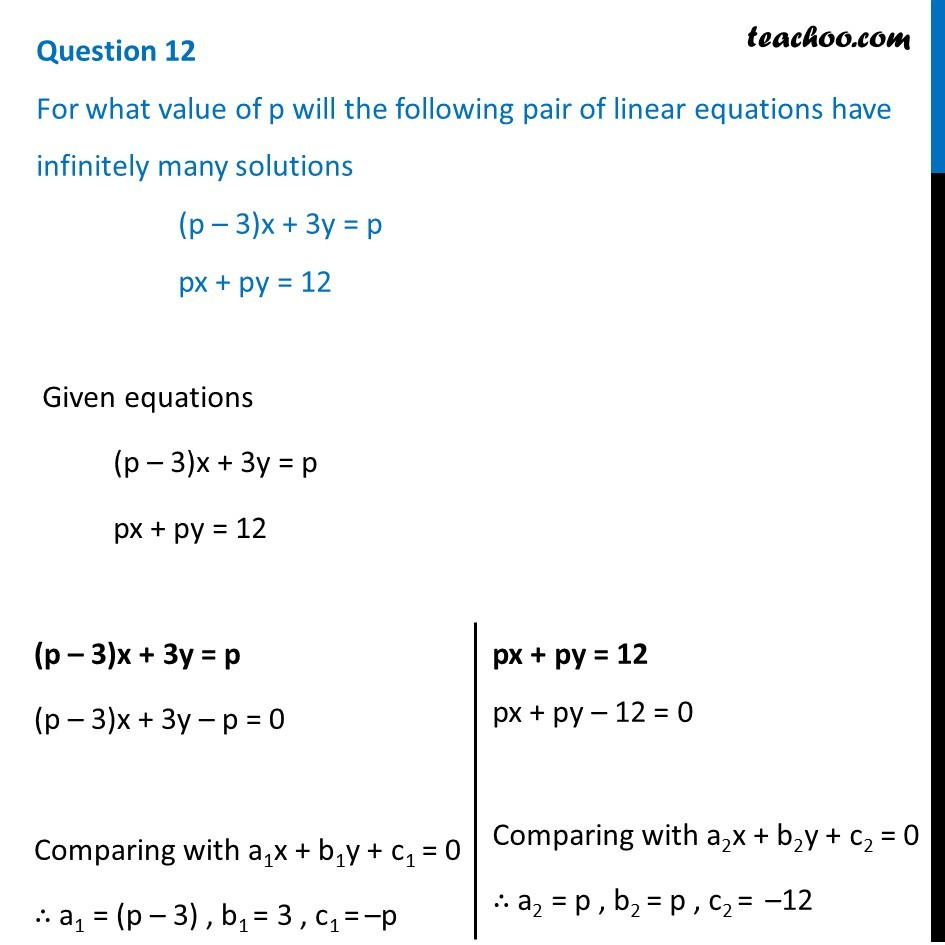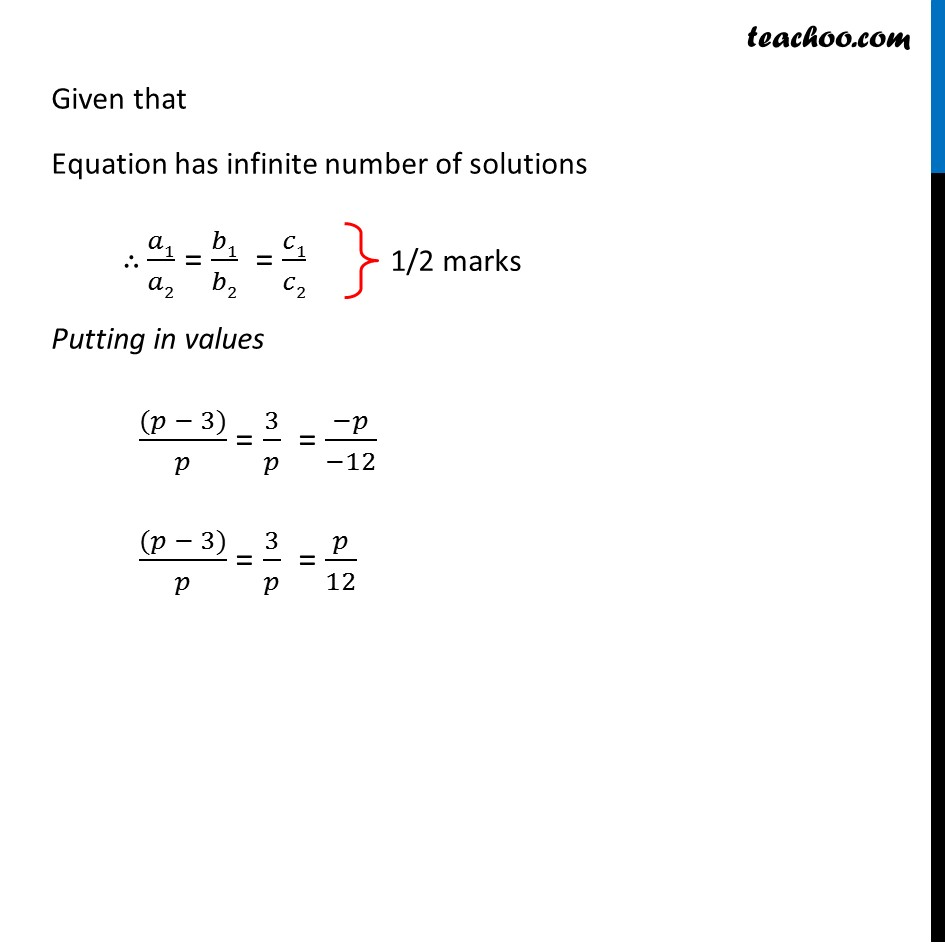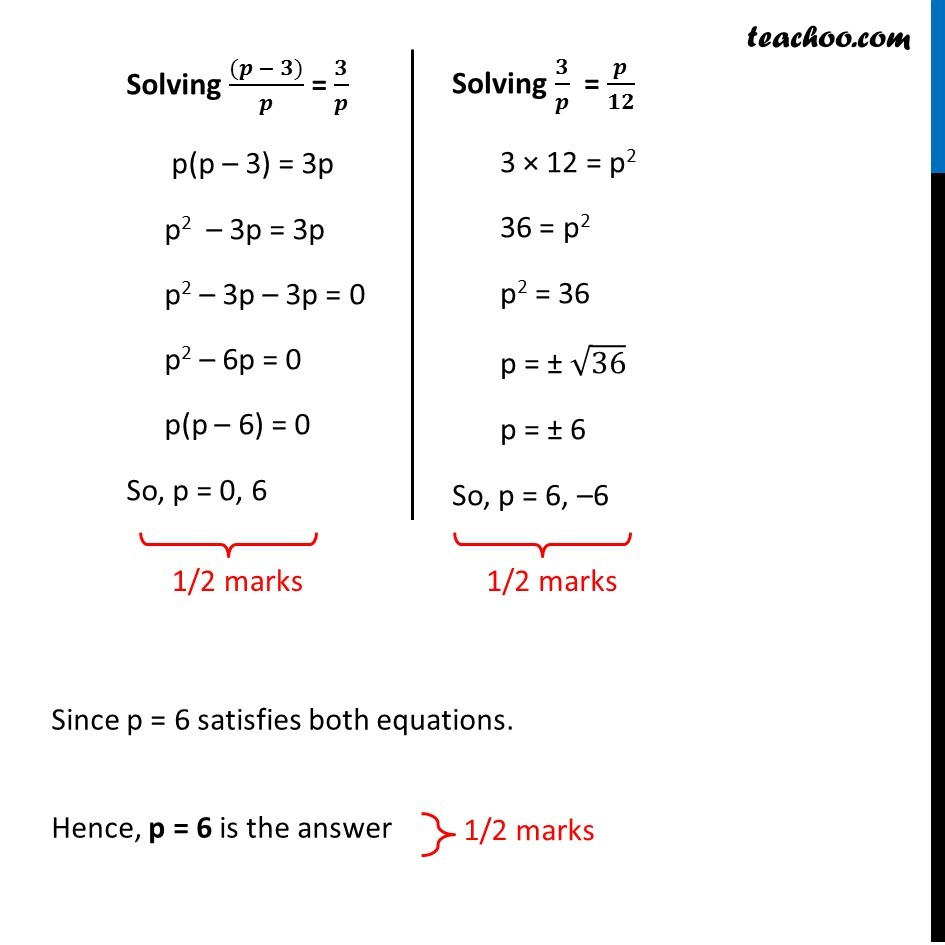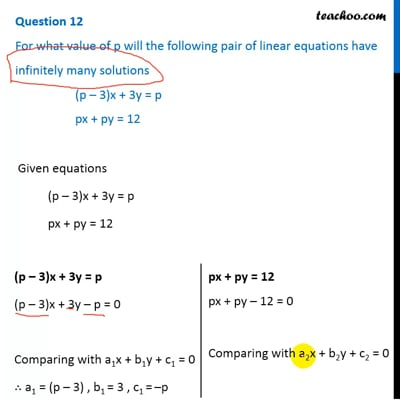CBSE Class 10 Sample Paper for 2019 Boards

Class 10
Solutions of Sample Papers for Class 10 Boards

Question 12

For what value of p will the following pair of linear equations have infinitely many solutions

(p – 3)x + 3y = p

px + py = 12This video is only available for Teachoo black users

### Transcript

Question 12 For what value of p will the following pair of linear equations have infinitely many solutions (p – 3)x + 3y = p px + py = 12 Given equations (p – 3)x + 3y = p px + py = 12 (p – 3)x + 3y = p (p – 3)x + 3y – p = 0 Comparing with a1x + b1y + c1 = 0 ∴ a1 = (p – 3) , b1 = 3 , c1 = –p px + py = 12 px + py – 12 = 0 Comparing with a2x + b2y + c2 = 0 ∴ a2 = p , b2 = p , c2 = –12 Given that Equation has infinite number of solutions ∴ 𝑎1/𝑎2 = 𝑏1/𝑏2 = 𝑐1/𝑐2 Putting in values ((𝑝 − 3))/𝑝 = 3/𝑝 = (−𝑝)/(−12) ((𝑝 − 3))/𝑝 = 3/𝑝 = 𝑝/12 1/2 marks Solving ((𝒑 − 𝟑))/𝒑 = 𝟑/𝒑 p(p – 3) = 3p p2 – 3p = 3p p2 – 3p – 3p = 0 p2 – 6p = 0 p(p – 6) = 0 So, p = 0, 6 1/2 marks Solving 𝟑/𝒑 = 𝒑/𝟏𝟐 3 × 12 = p2 36 = p2 p2 = 36 p = ± √36 p = ± 6 So, p = 6, –6 1/2 marks Since p = 6 satisfies both equations. Hence, p = 6 is the answer 1/2 marks